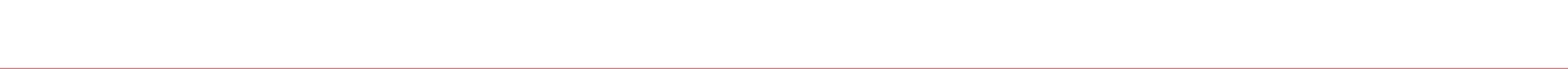Stock selection with classification tree based algorithms (Part II)

Chuan Shi   2016-11-15 本文章702阅读

1

Recap

In part I of this series, we introduced classification tree and its CART algorithm. The shortcoming of a classification tree is that it is sensitive to training data and therefore has high variance in its prediction to out-of-sample data. To reduce its variance, it is natural to employee multiple trees and average their results as a final prediction. In this regard, some advanced algorithms such as bagging, boosting, and random forest are all great examples. They are all ensemble learning meta algorithm. The term 'meta algorithm' means the algorithm of algorithms. They are techniques that can be applied to a single classification tree to improve the classification accuracy.

In today's article, we will talk about bagging, boosting, and random forest. Before that, let's introduce a prerequisite -- Bootstrapping.

2

Bootstrapping

2.1 Resample with replacement

Bootstrap (or bootstrapping) is term coined by Bardley Efron, and it is a key idea in statistic inference to perform computations on the data itself to estimate the variation of statistics that are themselves computed from the same data. To understand bootstrapping, let's start with another technique called resampling with replacement.

Here, 'with replacement' is the key. To explain it, think about the following example. Suppose a jar contains 10 balls labelled from 1 to 10, and we draw balls from the bag one at a time. Suppose we get No. 3 ball in the first draw. 'Resampling with replacement' requires we put it back into the jar before the next draw. As a consequence, in the second draw, we are equally likely to draw any of the 10 balls, including No. 3 ball. In contrast, there are many 'resampling without replacement' in our daily life, such as the 36/7 lottery and the World Cup draw. In those circumstances, the balls won't be put back once they are drawn from the pool.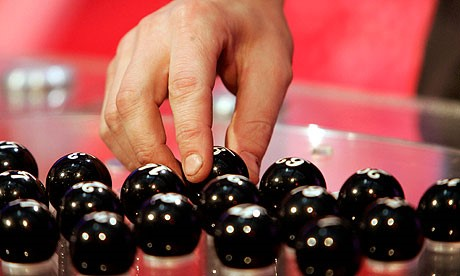2.2 Generate a large number of random resamples

Go back to the previous example where we have 10 balls. Suppose that we draw with replacement and get a resample of 10 balls, and their numbers are 3, 5, 6, 1, 1, 7, 9, 10, 2, 6 (i.e., ball No. 1 and No. 6 are drawn twice, while ball No. 4 and No. 8 are not chosen at all). This is an example of a bootstrap sample. By repeating the process above for many times, we can generate as many bootstrap samples as we want. As we will show below, doing this is a great deal.

2.3 Statistical meaning of bootstrapping

Bootstrapping is capable of measuring the error of the sample statistics when they are used as the estimates of the true yet unknown population statistics. Suppose that we would like to know the average height of all people all over the world. However, there are 7.4 billion people globally and it is impossible to meaure the height for each of them and then calculate the average. Instead, it is only feasible to analyze the issue using a random sample. Suppose we have a sample of 50,000 people randomly chosen and we know their heights. The sample mean is, say, 1.72 meters, which is a point estimate of the population mean. However, we don't know the error when it is used to estimate the population mean. This is because we only have one sample (of 50,000 people) but not any other samples from the population. Therefore, we cannot tell how sample mean varies around the population mean. Bootstrap is of great help in this situation.

Specifically, we take the sample at hand as our original data, and generate a large number (say 10,000) of bootstrap resample. From each resample we derive its average height and this gives us a distribution of the bootstrap mean. Bootstrap princple says we can use how bootstrap mean varies original sample mean as a good approximation of how original sample mean various the population mean.

We have a sample statistic u and we would like to use it to statistically infer the population statistic v. With bootstrapping we generate a large number of resamples and compute bootstrap statistic u* for each of them. They consititute the distribution of u* from which we can tell how u* varies around u. Bootstrap principle says the variation of u around v can be well-approximately by the variation of u* around u. Therefore, with the distribution of u* from bootstrapping, we can measure the error when u is used as an estimate of v.

3

Bagging

3.1 What's bagging?

Bagging is proposed by Breiman (1996a, 1996b). Bagging comes from Bootstrap aggregating, which conveys two key concepts, bootstrap and aggregate.

bootstrap:

Generate a large number of bootstrap samples from the original training data set. For each resample, grow a classification tree.

aggregate:

For each new sample, make predictions from all these trees and average their results according to the majority rule to find its final class label.

3.2 Why is bagging useful?

A classification tree is byitself unstable, since it is sensitive to training data. Small variation of features in the training data could lead to changes to tree structure, which in turn could give different predictions about the same sample. This means that a classification tree has large prediction variation.

The prediction error of a classification algorithm consists of bias and variation (Sutton 2005). Bagging takes advantage of aggregating of a large amount of classification trees to reduce the variation significantly. It is true that this may also increase the bias as a side effect. But since the magnitude of variation reduction is (much) bigger than that of bias increase, the error as a whole is reduced. For classification trees who usually have small bias but large variation, bagging is very effective to improve its accuracy.

In practice, Hastie et al. (2001) shows that 50 to 100 would be great candidates for number of bootstrap resamples (denoted by B). More trees than this rule-of-thumb will not significantly improve the accuracy anymore. Although making B large means that creating the classifier will take longer, some of the increased time requirement is offset by the fact that with bagging one does not have to use K-fold cross validation (as it is the case for growing a single tree) to select the right amount of complexity. When bagging, one can use the original sample as a test set. Alternatively, one could use out-of-bag estimates, letting the cases not selected for a particular bootstrap sample serve as independent test sample cases to use in the creation of the classifier from the bootstrap sample.

The following example illustrates the benefits of bagging. Suppose we have two-dimensional data and the subfigure on the left shows the population of two classes of data. Each point is represented by its coordinates (X1, X2) and its class is colored by red or green. The black circle represents the true decision boundary. Suppose we randomly extract 200 points from the population and use them to train a single classification tree, and the resulting decision boundary is shown in the middle subfigure. The difference between the true decision boundary and the boundary from learning this sample is very obvious, which means that the classification error of this single tree is huge. The right subfigure shows the results from bagging. Note that we still only have those 200 sample points, but after bootstrap resampling and aggregation, the final decision boundary from bagging is much smoother than that from the single tree, and it is closer to the true decision boundary. Bagging significantly improves the classification accuracy.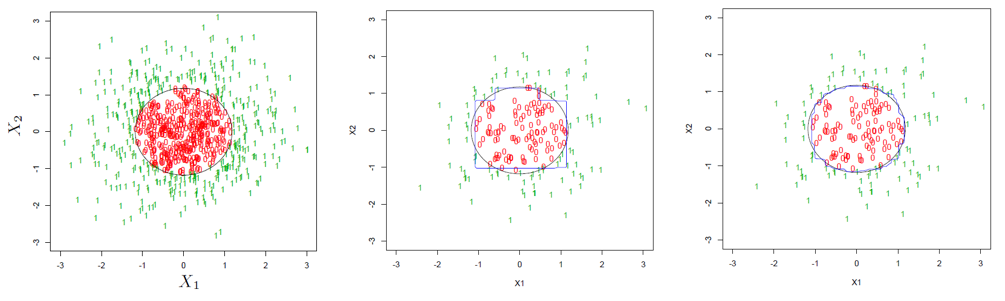4

Random forest

Random forest was proposed by Breiman (2001). It is similar to bagging and can be considered as an advanced version of bagging. Since bagging uses multiple trees, it is already a 'forest'. So, the difference between random forest and bagging is the randomness that the former brings to the process.
In the bagging technique, the bootstrap samples are all drawn from the same original sample data. As a consequence, they are still highly correlated. Breiman showed that the upper bound of the classification error is related to the correlation among the resamples. To further lower the error, one must try to reduce the correlation. This motivated him to propose random forest algorithm by brining randomness into bagging.

Specifically, the random forest algorithm does not differ from bagging when it constructs large collections of bootstrap samples by resampling. However, when training the classification tree for each resample, it introduces randomness into feature selection. When selecting the feature at a given split, it first randomly selects a subset of all candidate features, and then selects the best feature from the subset. If bagging considers only the randomness of samples (by bootstrapping), random forest takes into account both sample randomness and feature randomness, thus reducing the correlation between different classification trees.

5

Boosting

Boosting is another method of combining single trees. The motivation behind boosting is to answer the following question: can a group of week learns become a strong learner by ensemble learning?

A week learner can be a single tree or some other classifier that may only be slightly better than random guessing. A strong learner is the one with much higher prediction accuracy than a week learner. Since the algorithm turns a week learner to a strong learner, it is named boosting.

Freund and Schapire (1996) proposed AdaBoost. It iteratively creates trees using weighted version of the sample data, with the weights adaptively adjusted at each iteration to give increased weight to the cases which were misclassified on the previous step. As a result, those cases are more likely to be correctly classified in the new tree. The final predictions are obtained by weighting the results of the iteratively produced predictors.

We illustrate this process with a simple example below. Suppose our sample data belongs to two different classes of red and blue. First, we assign equal weights to all sample points, and use a weak classifier (i.e., a single tree) to classify them, and the decision boundary is represented by the dashed line. It can be seen that a red sample and two blue samples are misclassified. In the second iteration, the weights of these three points are increased (the weights of other points are therefore reduced), and then another tree is grown using the newly weighted sample data. At this time, two blue sample points are misclassified. In the third iteration, their weights are increased, and then a third tree is trained to obtain a new decision boundary. Repeat the process until the iteration reaches a certain number of times, such as M times, and this creates M week learners. For any new sample to be classified, the prediction results from these M weak classifiers are weighted according to their respective classification errors, and the weighted average becomes the final prediction of the new sample point.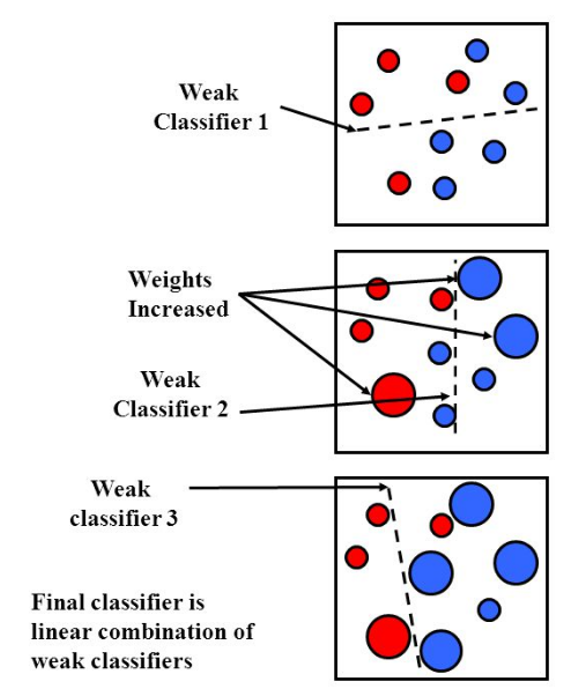As can be seen from the above description, at each step of the iteration, the new classification results tend to be more accurate in classifying previously misclassified samples. As a result, the decision boundaries of different trees vary considerably, resulting in low correlation among them. This is why this method could reduce the classification error effectively. Breiman also made a conjecture that AdaBoost can be seen as a special random forest. For specific mathematical expressions and procedures for the AdaBoost algorithm, please refer to Freund and Schapire (1996).

6

Comparing different algorithms

There is no doubt that bagging, random forest and boosting all improve the classification accuracy of a single tree. For the comparison among them, the random forest and the boosting method are effective in reducing the correlation between the different classification trees, and their effects are superior to the bagging method. The following figure shows the error comparison between bagging and AdaBoost on a certain classification problem. With the increased number of classification trees, their classification errors gradually converge, and the error of AdaBoost is obviously lower than that of bagging.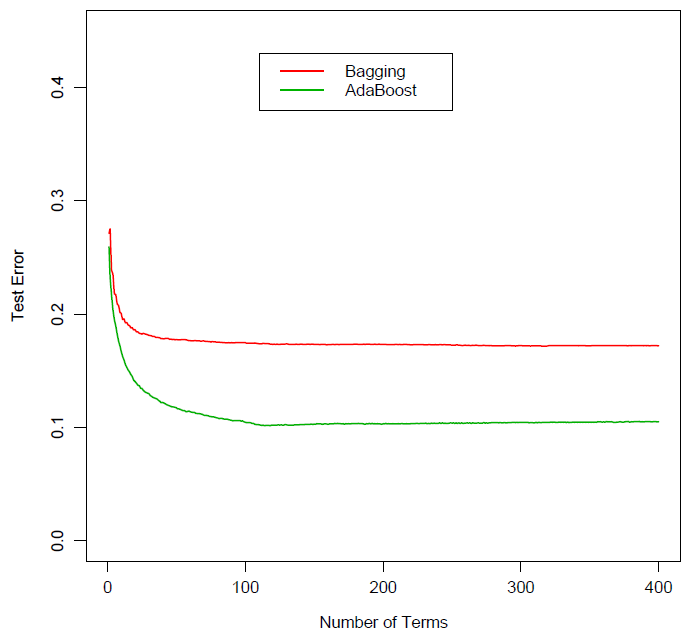However, there is still a debate over which one between random forest and boosting is better. Some scholars believe that boosting is better than random forest, while Breiman, the inventor of the latter, points out that random forest is superior than boosting. Judging from the results of a large number of industrial problems, these two methods are both excellent classification algorithms, and their effects on many problems are comparable to those of neural networks and support vector machines.

References

Breiman, L. (1996a). Bagging predictors. Machine Learning Vol. 24, 123–140.

Breiman, L. (1996b). Heuristics of instability and stabilization in model selection. Ann. Statist. Vol. 24, 2350–2383.

Breiman, L. (2001). Random forests. Machine Learning Vol. 45, 5–32.

Breiman, L., Friedman, J.H., Olshen, R.A., Stone, C.J. (1984). Classification and Regression Trees. Wadsworth, Pacific Grove, CA.

Freund, Y., Schapire, R. (1996). Experiments with a new boosting algorithm. In: Saitta, L. (Ed.), Machine Learning: Proceedings of the Thirteenth International Conference. Morgan Kaufmann, San Francisco, CA.

Hastie, T., Tibshirani, R., Friedman, J. (2001). The Elements of Statistical Learning: Data Mining, Inference, and Prediction. Springer-Verlag, New York.

Sutton, C. D. (2005). Classification and Regression Trees, Bagging, and Boosting. In Rao, C. R., Wegman, E. J., Solka, J. L. (Eds.), Handbook of Statistics 24: Data Mining and Data Visualization, Chapter 11.